https://doi.org/10.5194/bg-18-1619-2021
https://doi.org/10.5194/bg-18-1619-2021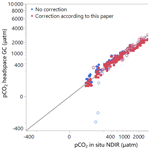# Technical note: CO2 is not like CH4 – limits of and corrections to the headspace method to analyse pCO2 in fresh water

Matthias Koschorreck, Yves T. Prairie, Jihyeon Kim, and Rafael Marcé
Abstract

Headspace analysis of CO2 frequently has been used to quantify the concentration of CO2 in fresh water. According to basic chemical theory, not considering chemical equilibration of the carbonate system in the sample vials will result in a systematic error. By analysing the potential error for different types of water and experimental conditions, we show that the error incurred by headspace analysis of CO2 is less than 5 % for typical samples from boreal systems which have low alkalinity (< 900 µmol L−1), with pH < 7.5, and high pCO2 (> 1000 µatm). However, the simple headspace calculation can lead to high error (up to 300 %) or even impossibly negative values in highly undersaturated samples equilibrated with ambient air, unless the shift in carbonate equilibrium is explicitly considered. The precision of the method can be improved by lowering the headspace ratio and/or the equilibration temperature. We provide a convenient and direct method implemented in an R script or a JMP add-in to correct CO2 headspace results using separately measured alkalinity.

Share
Dates
1 Introduction

The analysis of dissolved CO2 in water is an important basis for the assessment of the role of surface waters in the global carbon cycle (Raymond et al., 2013). Indirect methods like calculating CO2 from other parameters like alkalinity and pH (Lewis and Wallace, 1998; Robbins et al., 2010) are affected by considerable random and systematic errors (Golub et al., 2017) caused for example by dissolved organic carbon, which may result in significant overestimation of the CO2 partial pressure (pCO2) (Abril et al., 2015), or by pH measurement errors (Liu et al., 2020). Thus, direct measurement of CO2 is highly recommended, particularly in soft waters.

Headspace analysis is a standard method to analyse the concentration of dissolved gases in liquids (Kampbell et al., 1989). In principle, a liquid sample is equilibrated with a gaseous headspace in a closed vessel under defined temperature. The partial pressure of the gas in the headspace is analysed, in most cases either by gas chromatography or infra-red spectroscopy. The concentration of the dissolved gas in solution is then calculated by applying Henry's law after correction for the amount of gas transferred from the solution to the headspace.

In freshwater research this is the widely applied standard method to analyse the concentration of greenhouse gases such as CH4 and N2O (UNESCO/IHA, 2010). The method is handy, does not depend on sophisticated equipment in the field, and provides reliable results. Papers and protocols using this method have also been published to analyse dissolved CO2 concentrations in fresh waters (UNESCO/IHA, 2010; Cawley, 2018; Lambert and Fréchette, 2005). However, CO2 cannot be treated like CH4 because CO2 is in dynamic chemical equilibrium with other carbonate species in water while CH4 is not (Stumm and Morgan, 1981; Sander, 1999). Depending on the CO2 concentration and pH, reactions of the carbonate equilibrium will either produce or consume some CO2 in the sample vessel (Cole and Prairie, 2009). Although this is textbook knowledge and has been considered in some recent papers (Golub et al., 2017; Gelbrecht et al., 1998; Rantakari et al., 2015; Aberg and Wallin, 2014; Horn et al., 2017), and is standard practice in marine research (Dickson et al., 2007), a practical evaluation of the systematic error when applying simple headspace analysis to CO2 in typical fresh waters is missing, presumably because it is widely assumed that “the effect is likely small” (Hope et al., 1995). In this paper, we aim to quantify the error associated with the simple application of Henry's law on headspace CO2 data, present practical guidelines describing conditions under which the simple headspace analysis of CO2 can give acceptable results, and offer a convenient tool for the exact CO2 calculation that accounts for the carbonate equilibrium shifts in the sample equilibration vessel. The approach can also be used for correcting previous results obtained by simple headspace analysis of CO2 using additional information regarding the carbonate system (i.e. alkalinity or DIC), a procedure we tested on a set of field measurements where pCO2 was determined with independent methods (with and without headspace equilibration). Lastly, we evaluated how likely it is for this correction to be required when using a large dataset from 337 diverse Canadian lakes.

2 Methods

## 2.1 Theoretical considerations

If a water sample is equilibrated with a headspace initially containing a known pCO2 (zero in case N2 or another CO2-free gas is used), some CO2 is exchanged between water and headspace, resulting in an altered dissolved inorganic carbon (DIC) concentration in the water of the sample, thereby altering the equilibrium of the carbonate system in the water. Depending on partial pressure of CO2 in the water relative to the headspace gas prior to equilibration, some CO2 will either be produced from HCO${}_{\mathrm{3}}^{-}$ or converted to HCO${}_{\mathrm{3}}^{-}$. The exact amount will depend on temperature, pH, total alkalinity (TA), and the original pCO2 of the water sample. If a CO2-free headspace gas was applied, the vessel will finally contain more CO2 than before equilibration, and consequently simply applying Henry's law results in too high a pCO2 value. If an ambient-air headspace is applied, the error becomes negative in undersaturated samples and the calculated pCO2 an underestimate.

To calculate this error we implemented an R script that simulates the above-mentioned physical and chemical equilibration for a wide range of hypothetical pCO2, alkalinity, temperature, and headspace ratio (HR $={V}_{\mathrm{gas}}/{V}_{\mathrm{liquid}}$) values. As output, we then compared the corrected (for the chemical equilibrium shift) and non-corrected pCO2 values. All simulations were performed at 1 atm total pressure and results expressed as µatm.

## 2.2 Field data

$\begin{array}{}\text{(1)}& p{\mathrm{CO}}_{\mathrm{2}\phantom{\rule{0.125em}{0ex}}\mathrm{water}}=\frac{\begin{array}{c}\left({\mathrm{mCO}}_{\mathrm{2}\phantom{\rule{0.125em}{0ex}}\mathrm{After}\phantom{\rule{0.125em}{0ex}}\mathrm{eq}}×{K}_{\mathrm{hEq}}×P\right)\\ +\left\{\left(\frac{{V}_{\mathrm{gas}}}{{V}_{\mathrm{liquid}}}\right)×\left(\frac{{\mathrm{mCO}}_{\mathrm{2}\phantom{\rule{0.125em}{0ex}}\mathrm{After}\phantom{\rule{0.125em}{0ex}}\mathrm{eq}}-{\mathrm{mCO}}_{\mathrm{2}\phantom{\rule{0.125em}{0ex}}\mathrm{Before}\phantom{\rule{0.125em}{0ex}}\mathrm{eq}}}{{V}_{\mathrm{m}}}\right)\right\}\end{array}}{{K}_{\mathrm{h}\phantom{\rule{0.125em}{0ex}}\mathrm{Sample}}},\end{array}$

where mCO2 Before eq and mCO2 After eq are the CO2 mole fractions in the headspace before and after equilibrium (ppm) respectively, Kh Eq and Kh Sample are the gas solubility at the equilibration temperature and at the sampling temperature (Henry coefficient, Sander, 2015) (mol L−1 atm−1), P is pressure (atm), Vgas is the headspace volume, Vliquid is the sampled-water volume, and Vm is the molar volume (L mol−1) (UNESCO/IHA, 2010). Results from Eq. (1) are reported as pCO2 at 1 atm of barometric pressure and are corrected for ambient pressure at the time of sampling by multiplying with the in situ atmospheric pressure.

The difference between the headspace and NDIR method was divided by the pCO2 measured by the in situ NDIR analysis and expressed as percent (%) error. In addition, temperature and pH of the water were measured in situ by a CTD probe (Sea and Sun, Germany) or a portable pH meter (pH meter 913, Metrohm Ltd, Canada). In samples from Canada and Germany, TA was analysed by titration with 0.11 N HCl. In some systems, a single TA measurement was available for multiple dates and therefore assumes little temporal variability in the alkalinity of these systems. In the Malaysian samples, TA was derived from dissolved inorganic carbon (DIC) measurements and pH. Analysis of certified calibration gases showed that the analytical error of both the NDIR instrument and GC was < 0.37 % at 1000 ppm. Analysis of seven replicate samples by our GC-headspace method gave a standard deviation of 6 %. This includes all random errors due to sampling, sample handling, and analysis.

To demonstrate the effect of our correction procedure, we used data from 377 lakes for which we had complete ancillary data and precise headspace measurements of CO2 (< 5 % error between duplicates) obtained from the pan-Canadian Lake Pulse sampling programme (Fig. B1a; see Huot et al., 2019 for details).

3 Results and discussion

## 3.1 Simulations from chemical equilibrium

Applying a CO2-free gas as headspace always results in a positive error (overestimation of the real pCO2, Fig. 1a). If ambient air is applied as headspace, the error becomes negative in case of undersaturated samples (Fig. 1b). In general, the error tends to be lower if ambient air is used for headspace equilibration (Fig. 1b) compared to equilibration with CO2-free gas (Fig. 1a), except in undersaturated conditions. This is because less CO2 is exchanged between water and headspace during the equilibration procedure. The error will be below 5 % in supersaturated and low-alkalinity (< 900 µmol L−1) samples, which are typical for boreal regions. However, the error can be higher than 100 % if the samples are undersaturated. The magnitude of the error is predictable from pH. Because of the carbonate equilibrium reactions, high pH is necessarily accompanied by low pCO2 for a given alkalinity. Consequently, the error is large at high pH, while it is below 10 % at pH < 8 (headspace gas–liquid ratio of 1 : 1).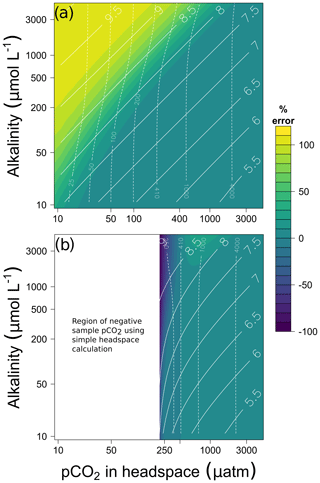Figure 1Error (%) when applying simple headspace calculations of pCO2 on hypothetical water samples of different alkalinity and pCO2 in the headspace after equilibration for (a) CO2-free gas headspace and (b) ambient-air headspace assuming a pressure of 1 atm. The resulting pH and pCO2 of the samples are depicted as full and dashed lines, respectively. The headspace ratio is 1 : 1, and the equilibration and field temperature is 20 C. Note the log scale in all axes. In panel (b) results for pCO2 in headspace after equilibration lower than 215 µatm are masked, because they would imply negative pCO2 in the sample.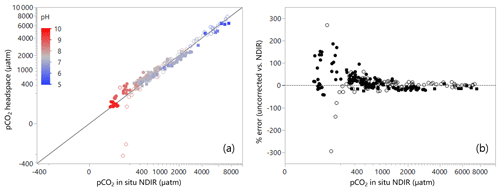Figure 2(a) Field data from 11 lakes, five reservoirs, and three streams in Germany, Canada, and Malaysia comparing pCO2 derived from simple headspace analysis with direct pCO2 measurements by NDIR analysis (pH colour coded). Note the cube-root scale in both axes. (b) Difference between the pCO2 derived from the simple headspace analysis and the direct pCO2 measurements by NDIR analysis expressed as error (%) as a function of the directly measured pCO2 by NDIR analysis. Note the cube-root scale in the x axis. Open-circle symbols: ambient-air headspace; closed-circle symbols: CO2-free gas headspace; and closed-square symbols: pre-measured-CO2 gas (between 150 to 250 ppm) headspace applied.

Our field dataset is consistent with the theoretical predictions. While the fit between the simple headspace calculation and NDIR values over the whole range of values can be considered adequate overall (Fig. 2a, R2=0.92), it is clear that the deviations can become very large (up to about 300 %), particularly at water pCO2 values < 600 µatm (Fig. 2b). As expected from the simulations, the error in undersaturated samples was positive when using CO2-free gas as headspace and negative (sometimes impossible negative results) when using ambient air (Fig. 2b). The error became negligible at pCO2 above 1000 µatm (Fig. 2b). Data scatter was considerable as was observed previously (Johnson et al., 2010), most probably because the analytical error of the applied methods was often in the same range as the absolute difference between both methods.

## 3.2 Error magnitude depends on the experimental procedure

The maximum error depends on how much CO2 is exchanged between water and headspace. The more gas is exchanged between water and headspace the higher the error is. Thus, the error increases with decreasing solubility coefficient or HR. In high-alkalinity samples, the error can be significantly reduced by using a smaller headspace-to-water ratio (Fig. 3). By lowering the headspace ratio from 1 to 0.2 at 20 C, the error can be reduced from about 50 % to about 10 %.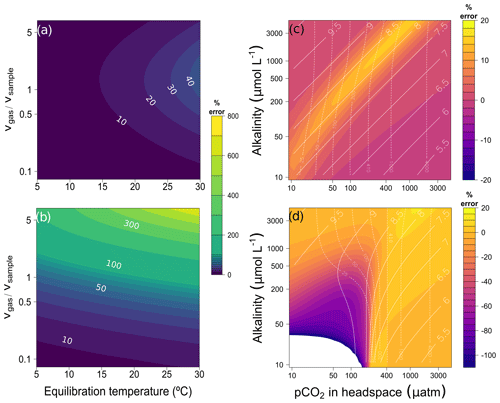Figure 3Error (%) when applying simple headspace calculation depending on headspace ratio and equilibration temperature for (a) 100 µmol L−1 and (b) 1000 µmol L−1 alkalinity. Panels (a) and (b) were constructed using highly undersaturated conditions (headspace pCO2= 50 µatm after equilibration and field water temperature of 20 C). The values of some isolines are added for reference. (c) Error (%) applying our complete headspace method when the alkalinity value supplied for calculations is off the real alkalinity of the sample by +50 %. The results are for hypothetical water samples of different alkalinity and pCO2 in the headspace after equilibration using CO2-free gas headspace, a headspace ratio of 1 : 1, and an equilibration and field temperature of 20 C. (d) Like panel (c) but with air headspace. All calculations assume a pressure of 1 atm.

Since solubility of CO2 depends on temperature, the equilibration temperature also affects headspace equilibration. Due to lower solubility at higher temperature, more gas evades into the headspace, and thus the error increases with increasing temperature (Fig. 3a, b). At a HR of 1, the error increases from 97 % at 20 C to 111 % at 25 C in a high (1 mmol L−1) alkalinity sample. Thus, the error can be significantly reduced by lowering the equilibration temperature. A possible way to take advantage of this is to perform headspace equilibration at in situ temperature in the field, as has been done in several studies. If in situ water temperature is lower than typical laboratory temperature, the error is thereby reduced. However, care must be taken to make sure that the exact equilibration temperature is known. For example, an error of 1 C in the equilibration temperature results in a 2 % different pCO2 value (TA = 1 mmol L−1, pCO2= 1000 µatm, HR = 1) (Fig. A1a). Both ambient air and N2 can be used as headspace gas. Using N2, however, eliminates the error associated with the exact quantification of pCO2 Before. Using the same example, an unlikely error of 100 ppm in the headspace gas (mCO2 Before eq) results in a 6.4 % different pCO2 result (Fig. A1b).

CO2 reactivity with water would not cause a problem for headspace analysis if the reaction kinetics were slow compared to physical headspace equilibration. The slowest reaction of the carbonate system is the hydration of CO2, which has a first-order rate constant of 0.037 s−1 (Soli and Byrne, 2002), so that chemical equilibration of CO2 in water is in the range of seconds (Zeebe and Wolf-Gladrow, 2001; Schulz et al., 2006). This means that chemical equilibrium reactions are faster than physical headspace equilibration, and the chemical system can be assumed to always be in equilibrium. Thus, the reactions of the carbonate system have to be fully considered in headspace analysis of CO2.

## 3.4 Correction of CO2 headspace data

If other information regarding the carbonate system of the sample is known (alkalinity or DIC), one can correct for the bias induced by simple headspace calculations. A procedure to correct headspace CO2 data using pH and alkalinity is already available in the standard operating procedure (SOP) no. 4 in Dickson et al. (2007) for marine samples and could be adapted to freshwater samples as well. For convenience, we provide here a modified procedure when the alkalinity of the sample is known by introducing an analytical solution to the equilibrium problem (iterative in SOP no. 4) and by using dissociation constants that may be more appropriate to fresh waters. The procedure essentially involves estimating the exact pH of the equilibrium solution before and after equilibration. If the alkalinity of the sample is known, the pH (−log 10[H+]) of the aqueous solution after equilibration can be obtained by finding the roots of the third-order polynomial:

$\begin{array}{}\text{(2)}& \begin{array}{rl}\mathrm{0}& =\left[{\mathrm{H}}^{+}{\right]}^{\mathrm{3}}+\mathrm{TA}\cdot \left[{\mathrm{H}}^{+}{\right]}^{\mathrm{2}}-\left(\left[{\mathrm{CO}}_{\mathrm{2}}\right]{K}_{\mathrm{1}}+{K}_{\mathrm{w}}\right)\left[{\mathrm{H}}^{+}\right]\\ & -\mathrm{2}{K}_{\mathrm{1}}{K}_{\mathrm{2}}\left[{\mathrm{CO}}_{\mathrm{2}}\right],\end{array}\end{array}$

where $\left[{\mathrm{CO}}_{\mathrm{2}}\right]=p{\mathrm{CO}}_{\mathrm{2}}\cdot {K}_{\mathrm{h}\phantom{\rule{0.125em}{0ex}}\mathrm{Eq}}$ and from which one can obtain the ionization fraction for CO2 (${\mathit{\alpha }}_{{\mathrm{CO}}_{\mathrm{2}}}$) as

$\begin{array}{}\text{(3)}& {\propto }_{{\mathrm{CO}}_{\mathrm{2}}}=\frac{\mathrm{1}}{\mathrm{1}+\frac{{K}_{\mathrm{1}}}{\left[{\mathrm{H}}^{+}\right]}+\frac{{K}_{\mathrm{1}}{K}_{\mathrm{2}}}{\left[{\mathrm{H}}^{+}{\right]}^{\mathrm{2}}}},\end{array}$

where K1 and K2 are the temperature-dependent equilibrium constants for the dissociation reactions for bicarbonates and carbonates, respectively (Millero, 1979), and for estuarine conditions (Millero, 2010), as amended in Orr et al. (2015). Kw is the dissociation constant of water into H+ and OH (Dickson and Riley, 1979). The total DIC contained in the original sample (DICorig) can then be calculated as

$\begin{array}{}\text{(4)}& {\mathrm{DIC}}_{\mathrm{orig}}=\frac{{\mathrm{CO}}_{\mathrm{2}}}{{\mathit{\alpha }}_{{\mathrm{CO}}_{\mathrm{2}}}}+\left({\mathrm{CO}}_{\mathrm{2}\phantom{\rule{0.125em}{0ex}}\mathrm{HS}\phantom{\rule{0.125em}{0ex}}\mathrm{after}}-{\mathrm{CO}}_{\mathrm{2}\phantom{\rule{0.125em}{0ex}}\mathrm{HS}\phantom{\rule{0.125em}{0ex}}\mathrm{before}}\right),\end{array}$

where CO2 is the amount of CO2 in the equilibrated water (mol), and CO2 HS after and CO2 HS before are the amount of CO2 in the headspace after and before equilibration (mol). Given the DIC concentration of the original solution from Eq. (4) ([DIC] = DIC${}_{\mathrm{orig}}/{V}_{\mathrm{liquid}}$), the pH of this solution prior to equilibration can be obtained by finding the roots of the fourth-order polynomial,

$\begin{array}{}\text{(5)}& \begin{array}{rl}\mathrm{0}& =\left[{\mathrm{H}}^{+}{\right]}^{\mathrm{4}}+\left(\mathrm{TA}+{K}_{\mathrm{1}}\right)\cdot \left[{\mathrm{H}}^{+}{\right]}^{\mathrm{3}}\\ & +\left(\mathrm{TA}\cdot {K}_{\mathrm{1}}-{K}_{\mathrm{w}}+{K}_{\mathrm{1}}{K}_{\mathrm{2}}-{\left[\mathrm{DIC}\right]}_{\mathrm{orig}}{K}_{\mathrm{1}}\right)\cdot {\left[{\mathrm{H}}^{+}\right]}^{\mathrm{2}}\\ & +\left({K}_{\mathrm{1}}{K}_{\mathrm{2}}\cdot \mathrm{TA}-{K}_{\mathrm{1}}{K}_{\mathrm{w}}-\mathrm{2}{\left[\mathrm{DIC}\right]}_{\mathrm{orig}}{K}_{\mathrm{1}}{K}_{\mathrm{2}}\right)\cdot \left[{\mathrm{H}}^{+}\right]\\ & -{K}_{\mathrm{1}}{K}_{\mathrm{2}}{K}_{\mathrm{w}},\end{array}\end{array}$

to then estimate the corresponding ionization fraction ${\mathit{\alpha }}_{{\mathrm{CO}}_{\mathrm{2}}}^{\prime }$ as in Eq. (3) above and calculate the original pCO2 of the sample as

$\begin{array}{}\text{(6)}& p{\mathrm{CO}}_{\mathrm{2}}=\frac{{\mathit{\alpha }}_{{\mathrm{CO}}_{\mathrm{2}}}^{\prime }\cdot {\left[\mathrm{DIC}\right]}_{\mathrm{orig}}}{{K}_{\mathrm{h}\phantom{\rule{0.125em}{0ex}}\mathrm{Sample}}},\end{array}$

where Kh Sample is determined for the water temperature during field sample collection (for simplicity, the equations above assume 1 atm pressure). We applied the above correction procedure to our samples, where pCO2 was measured in several samples using both headspace and in situ NDIR methods together with measured alkalinity data. Figure 4 shows that the corrected values matched the in situ NDIR values nearly perfectly (r2=0.98), whereas the simple headspace calculations resulted, as expected, in significant underestimation for undersaturated conditions, particularly for samples equilibrated with ambient air.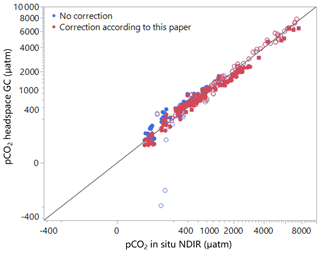Figure 4Comparison of uncorrected and corrected data with direct pCO2 measurements by NDIR analysis. Note the cube-root scale in both axes. Open-circle symbols: ambient-air headspace; closed-circle symbols: CO2-free gas headspace; and closed-square symbols: pre-measured-CO2 gas (between 150 to 250 ppm) headspace applied.

We examined the sensitivity of the correction procedure to the precision of the alkalinity measurements and found that the error associated with alkalinity determination does not severely impact the final pCO2 estimate when using N2 as a headspace gas. For example, the error in the corrected pCO2 values is always below 20 % even when the alkalinity is known only to within 50 % error (Fig. 3c). However, more precise alkalinity values are required when using ambient air as a headspace gas in undersaturated conditions (Fig. 3d).

Lastly, our simulations (Figs. 2 and 4) provide a complete analysis of the effects of the environmental and methodological conditions on the error incurred when using the simple headspace technique for estimating pCO2. However, they do not assess how often such problematic conditions occur in inland water systems. To address this question, we applied our correction procedure to a dataset from 377 Canadian lakes (Huot et al., 2019). These results show a significant deviation between corrected and uncorrected values, particularly in lakes with high alkalinity (> 900 µmol L−1, Fig. B1b), and ignoring the correction would have resulted in errors > 20 % in about 47 % of the data. Furthermore, our analysis illustrates how a larger headspace ratio significantly exacerbates the magnitude of the error (Fig. B1b).

The correction calculations have been implemented in an R script and, for a user-friendly interface, as a JMP add-in (or JSL script) (https://github.com/icra/headspace, last access: 3 March 2021). Roots of the polynomials (Eqs. 2 and 5) can be solved using either standard analytical formulas or by iterative algorithms. For the analytical solution, our script uses a combined form of the computational steps described in Zwillinger (2018) for both the cubic and quartic polynomials to find their first real roots. Analytical solutions are faster than iterative algorithms but can suffer small numerical instabilities (SD  1 ppm) in extreme situations (alkalinity > 4000 µmol L−1 and pCO2< 100 ppm) due to limitations inherent to double-precision numerical calculations. The provided scripts consider the barometric pressure and thus allow calculation of pCO2 as well as CO2 concentration (µmol L−1) for in situ conditions.

4 Conclusions

The headspace method has been used in several studies about CO2 fluxes from surface waters. Our error analysis shows that the usual headspace method can be used (error < 5 %) if the pH is below 7.5 or pCO2 is above 1000 µatm (TA < 900 µmol L−1, air headspace), a typical situation in most boreal systems. However, the standard headspace method introduces large errors and cannot be used reliably for undersaturated samples, which are typical of eutrophic or low-DOC systems. In all other cases, not accounting for the chemical equilibrium shift leads to a systematic overestimation. The magnitude of the error can be reduced by increasing the water–headspace ratio or lowering the equilibration temperature. The magnitude of that error can be roughly estimated from Fig. 1. If alkalinity is known, pCO2 obtained from headspace equilibration can be corrected by the provided scripts. We therefore recommend to always measure alkalinity if the headspace method is to be used for pCO2 determinations. The procedure can also be used to correct historical pCO2 data. Our field data showed that the correction works well even in highly undersaturated conditions and is not very sensitive to the precise determination of alkalinity if N2 is used as a headspace gas. The precision of the corrected pCO2 is similar to that obtained from direct pCO2 measurement using a field NDIR analyser coupled to an online equilibrator (Cole and Prairie, 2009; Yoon et al., 2016).

Appendix A: Sensitivity analysis equilibration temperature and CO2 Beforeeq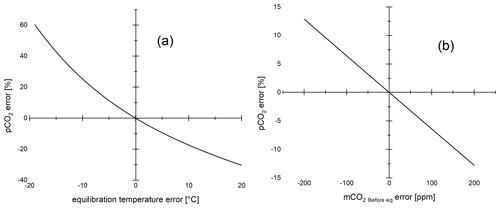Figure A1Error for a hypothetical sample with CO2 Before eq= 400 ppm, CO2 after eq= 1000 ppm, equilibration temperature of 20 C, and HR = 1 (a) depending on error in equilibration temperature and (b) depending on error in initial headspace gas composition.

Appendix B: Application of our correction to a large Canadian dataset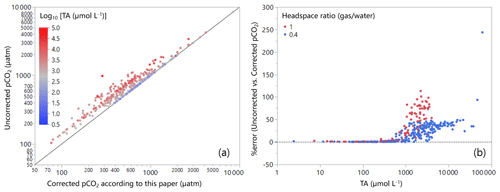Figure B1Field data from 377 lakes across Canada (a) for comparing pCO2 derived from simple headspace calculation with that from the corrected headspace calculation according to this paper (Log10 [TA (µmol L−1)] colour coded). (b) Difference between the uncorrected and corrected pCO2 expressed as error (%) as a function of TA (µmol L−1) (the headspace ratio colour coded). Note that CO2-free gas was used for headspace, and TA values were derived from DIC measurement and pH. More information about the dataset can be found in Huot et al. (2019).

Code availability

All codes are publicly available at https://github.com/icra/headspace (Marcé, 2020) and https://doi.org/10.5281/zenodo.4577401 (Marcé, 2021).

Data availability

All data can be found in the Supplement.

Supplement

Author contributions

All authors conceived the story, performed calculations, and wrote the manuscript. JK, YTP, and RM wrote codes. MK and JK contributed field data.

Competing interests

The authors declare that they have no conflict of interest.

Acknowledgements

We thank Philipp Keller, Peifang Leng, Lelaina Teichert, and Cynthia Soued for sharing additional field data as well as Alo Laas for logistic support. We thank Miitta Rantakari, Marcus Wallin, and Pascal Bodmer for stimulating discussions as well as Bertram Boehrer for commenting on the manuscript. We also thank Matt T. Trentman, Joanna R. Blaszczak and Robert O. Hall Jr. for an independent check of our calculations during the public discussion of the manuscript. Rafael Marcé participated through the project C-HYDROCHANGE, funded by the Spanish Agencia Estatal de Investigación (AEI) and Fondo Europeo de Desarrollo Regional (FEDER) under the contract FEDER-MCIU-AEI/CGL2017-86788-C3-2-P. Rafael Marcé acknowledges the support from the Economy and Knowledge Department of the Catalan Government through the Consolidated Research Group (ICRA-ENV 2017 SGR 1124), as well as from the CERCA programme. Jihyeon Kim and Yves T. Prairie were funded by the Natural Sciences and Engineering Research Council of Canada. This is a contribution to the UNESCO Chair in Global Environmental Change.

Financial support

This research has been supported by the Spanish Agencia Estatal de Investigación (AEI) and Fondo Europeo de Desarrollo Regional (FEDER) (grant no. FEDER-MCIU-AEI/CGL2017-86788-C3-2-P) and the Economy and Knowledge Department of the Catalan Government (grant no. ICRA-ENV 2017 SGR 1124).

The article processing charges for this open-access
publication were covered by a Research
Centre of the Helmholtz Association.

Review statement

This paper was edited by Peter Landschützer and reviewed by Matt Trentman and two anonymous referees.

References

Aberg, J. and Wallin, M. B.: Evaluating a fast headspace method for measuring DIC and subsequent calculation of PCO2 in freshwater systems, Inland Waters, 4, 157–166, 2014.

Abril, G., Bouillon, S., Darchambeau, F., Teodoru, C. R., Marwick, T. R., Tamooh, F., Ochieng Omengo, F., Geeraert, N., Deirmendjian, L., Polsenaere, P., and Borges, A. V.: Technical Note: Large overestimation of pCO2 calculated from pH and alkalinity in acidic, organic-rich freshwaters, Biogeosciences, 12, 67–78, https://doi.org/10.5194/bg-12-67-2015, 2015.

Cawley, K. M.: neonDissGas: Calculates Dissolved CO2, CH4, and N2O Concentrations in Surface Water, in: R-package, available at: https://github.com/NEONScience/NEON-dissolved-gas/tree/master/neonDissGas (last access: 3 March 2021), 2018.

Cole, J. J. and Prairie, Y. T.: Dissolved CO2, in: Encyclopedia of Inland Waters, Elsevier, available at: https://www.sciencedirect.com/referencework/9780123706263/encyclopedia-of-inland-waters#book-info (last access: 3 March 2021), 2009.

Dickson, A. G. and Riley, J. P.: The estimation of acid dissociation constants in seawater media from potentionmetric titrations with strong base. I. The ionic product of water – Kw, Mar. Chem., 7, 89–99, https://doi.org/10.1016/0304-4203(79)90001-X, 1979.

Dickson, A. G., Sabine, C. L., and Christian, J. R. (Eds.): Guide to best practices for ocean CO2 measurements, PICES special publication 3, 191 pp., available at: https://www.ncei.noaa.gov/access/ocean-carbon-data-system/oceans/Handbook_2007.html (last access: 3 March 2021), 2007.

Gelbrecht, J., Fait, M., Dittrich, M., and Steinberg, C.: Use of GC and equilibrium calculations of CO2 saturation index to indicate whether freshwater bodies in north-eastern Germany are net sources or sinks for atmospheric CO2, Fresen. J. Anal. Chem., 361, 47–53, https://doi.org/10.1007/s002160050832, 1998.

Golub, M., Desai, A. R., McKinley, G. A., Remucal, C. K., and Stanley, E. H.: Large Uncertainty in Estimating pCO2 From Carbonate Equilibria in Lakes, J. Geophys. Res.-Biogeo., 122, 2909–2924, https://doi.org/10.1002/2017jg003794, 2017.

Hope, D., Dawson, J. J. C., Cresser, M. S., and Billett, M. F.: A Method for Measuring Free CO2 in Upland Streamwater Using Headspace Analysis, J. Hydrol., 166, 1–14, https://doi.org/10.1016/0022-1694(94)02628-O, 1995.

Horn, C., Metzler, P., Ullrich, K., Koschorreck, M., and Boehrer, B.: Methane storage and ebullition in monimolimnetic waters of polluted mine pit lake Vollert-Sued, Germany, Sci. Total Environ., 584–585, 1–10, https://doi.org/10.1016/j.scitotenv.2017.01.151, 2017.

Huot, Y., Brown, C. A., Potvin, G., Antoniades, D., Baulch, H. M., Beisner, B. E., Bélanger, S., Brazeau, S., Cabana, H., Cardille, J. A., del Giorgio, P. A., Gregory-Eaves, I., Fortin, M.-J., Lang, A. S., Laurion, I., Maranger, R., Prairie, Y. T., Rusak, J. A., Segura, P. A., Siron, R., Smol, J. P., Vinebrooke, R. D., and Walsh, D. A.: The NSERC Canadian Lake Pulse Network: A national assessment of lake health providing science for water management in a changing climate, Sci. Total Environ., 695, 133668, https://doi.org/10.1016/j.scitotenv.2019.133668, 2019.

Johnson, M. S., Billett, M. F., Dinsmore, K. J., Wallin, M., Dyson, K. E., and Jassal, R. S.: Direct and continuous measurement of dissolved carbon dioxide in freshwater aquatic systems – method and applications, Ecohydrology, 3, 68–78, https://doi.org/10.1002/eco.95, 2010.

Kampbell, D., Wilson, J. T., and Vandegrift, S.: Dissolved oxygen and methane in water by a GC headspace equilibration technique, Int. J. Environ. Anal. Chem., 36, 249–257, 1989.

Lambert, M. and Fréchette, J.-L.: Analytical Techniques for Measuring Fluxes of CO2 and CH4 from Hydroelectric Reservoirs and Natural Water Bodies, in: Greenhouse Gas Emissions – Fluxes and Processes, edited by: Tremblay, V., Roehm, Garneau, Springer-Verlag, Berlin, Heidelberg, Germany, 37–60, 2005.

Lewis, E. and Wallace, D. W. R.: Program developed for CO2 system calculations. Carbon dioxide information analysis center, Oak Ridge National Laboratory, U.S. Department of Energy, Oak Ridge, Tennessee, USA, 1998.

Liu, S., Butman, D. E., and Raymond, P. A.: Evaluating CO2 calculation error from organic alkalinity and pH measurement error in low ionic strength freshwaters, Limnol. Oceanogr. Methods, 18, 606–622, https://doi.org/10.1002/lom3.10388, 2020.

Marcé, R.: Rheadspace.R, R Skript, GitHub, available at: https://github.com/icra/headspace (last access: 3 March 2021), 2020.

Millero, F. J.: Thermodynamics of the Carbonate System in Seawater, Geochim. Cosmochim. Ac., 43, 1651–1661, 1979.

Millero, F. J.: Carbonate constants for estuarine waters, Mar. Freshwater Res., 61, 139–142, https://doi.org/10.1071/MF09254, 2010.

Orr, J. C., Epitalon, J.-M., and Gattuso, J.-P.: Comparison of ten packages that compute ocean carbonate chemistry, Biogeosciences, 12, 1483–1510, https://doi.org/10.5194/bg-12-1483-2015, 2015.

Rantakari, M., Heiskanen, J., Mammarella, I., Tulonen, T., Linnaluoma, J., Kankaala, P., and Ojala, A.: Different Apparent Gas Exchange Coefficients for CO2 and CH4: Comparing a Brown-Water and a Clear-Water Lake in the Boreal Zone during the Whole Growing Season, Environ. Sci. Technol., 49, 11388–11394, https://doi.org/10.1021/acs.est.5b01261, 2015.

Raymond, P. A., Hartmann, J., Lauerwald, R., Sobek, S., McDonald, C., Hoover, M., Butman, D., Striegl, R., Mayorga, E., Humborg, C., Kortelainen, P., Durr, H., Meybeck, M., Ciais, P., and Guth, P.: Global carbon dioxide emissions from inland waters, Nature, 503, 355–359, https://doi.org/10.1038/Nature12760, 2013.

Robbins, L., Hansen, M., Kleypas, J., and Meylan, S.: CO2calc: A user-friendly seawater carbon calculator for Windows, Mac OS X, and iOS (iPhone), US Geological Survey, available at: https://pubs.usgs.gov/of/2010/1280/ (last access: 4 March 2021), 2010.

Sander, R.: Modeling atmospheric chemistry: Interactions between gas-phase species and liquid cloud/aerosol particles, Surv. Geophys., 20, 1–31, https://doi.org/10.1023/A:1006501706704, 1999.

Sander, R.: Compilation of Henry's law constants (version 4.0) for water as solvent, Atmos. Chem. Phys., 15, 4399–4981, https://doi.org/10.5194/acp-15-4399-2015, 2015.

Schulz, K. G., Riebesell, U., Rost, B., Thoms, S., and Zeebe, R. E.: Determination of the rate constants for the carbon dioxide to bicarbonate inter-conversion in pH-buffered seawater systems, Mar. Chem., 100, 53–65, https://doi.org/10.1016/j.marchem.2005.11.001, 2006.

Soli, A. L. and Byrne, R. H.: CO2 system hydration and dehydration kinetics and the equilibrium CO2$/$ H2CO3 ratio in aqueous NaCl solution, Mar. Chem., 78, 65–73, https://doi.org/10.1016/S0304-4203(02)00010-5, 2002.

Stumm, W. and Morgan, J. J.: Aquatic chemistry, Wiley, New York, USA, 1981.

UNESCO/IHA: GHG Measurement Guidlines for Freshwater Reservoirs, UNESCO, 138, The International Hydropower Association (IHA), London, UK, 2010.

Yoon, T. K., Jin, H., Oh, N.-H., and Park, J.-H.: Technical note: Assessing gas equilibration systems for continuous pCO2 measurements in inland waters, Biogeosciences, 13, 3915–3930, https://doi.org/10.5194/bg-13-3915-2016, 2016.

Zeebe, R. E. and Wolf-Gladrow, D.: CO2 in seawater: equilibrium, kinetics, isotopes, Elsevier oceanography Series, 65, edited by: Halpern, D., Elsevier, Amsterdam, the Netherlands, 2001.

Zwillinger, D.: CRC Standard Mathematical Tables and Formulas, Chapman and Hall/CRC, Boca Raton, USA, 872 pp., 2018.